Related Articles

# Join Multiple Tables Using Inner Join

• Last Updated : 27 May, 2021

To retrieve data from the single table we use SELECT and PROJECTION operations but to retrieve data from multiple tables we use JOINS in SQL. There are different types of JOINS in SQL. In this article, only operations on inner joins in MSSQL are discussed.

Inner Join is the method of retrieval of data from multiple tables based on a required condition and necessary conditions are that there must be common columns or matched columns between the two tables of the database and the data types of columns must be the same.

Let us see how to join two tables and three tables using Inner Join in MSSQL step-by-step.

### Creating a database :

Creating a database GeeksForGeeks by using the following SQL query as follows.

` CREATE DATABASE GeeksForGeeks;`### Using the database :

Using the database student using the following SQL query as follows.

`USE GeeksForGeeks;`Creating three tables student, course, and lecturer with SQL query as follows:

```CREATE TABLE student
( stu_id varchar(10),
stu_name varchar(20),
course_id varchar(10),
branch varchar(20),
lecturer_id varchar(10)
);```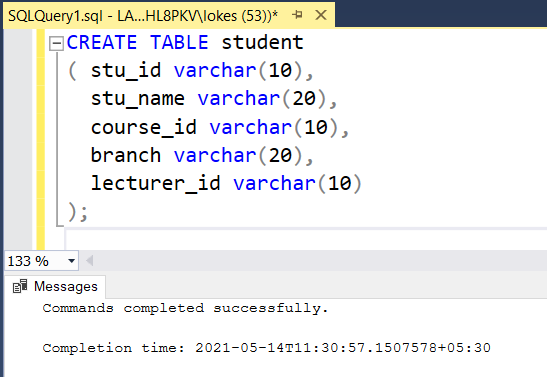```CREATE TABLE course
(
course_id varchar(10),
course_name varchar(20)
);```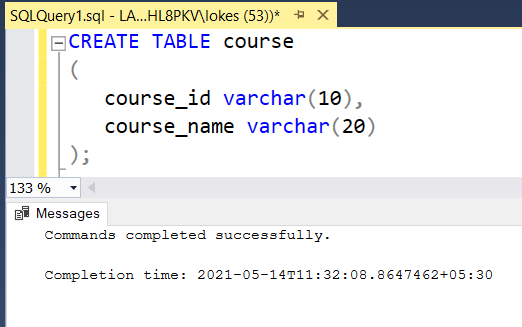```CREATE TABLE lecturer
(
lecturer_id varchar(10),
lecturer_name varchar(20)
);```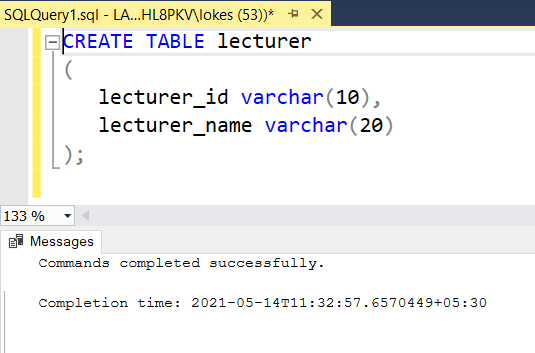### Verifying the database :

To view the description of the three tables in the database GeeksForGeeks using the following SQL query as follows.

```EXEC sp_columns student;
EXEC sp_columns course;
EXEC sp_columns lecturer;```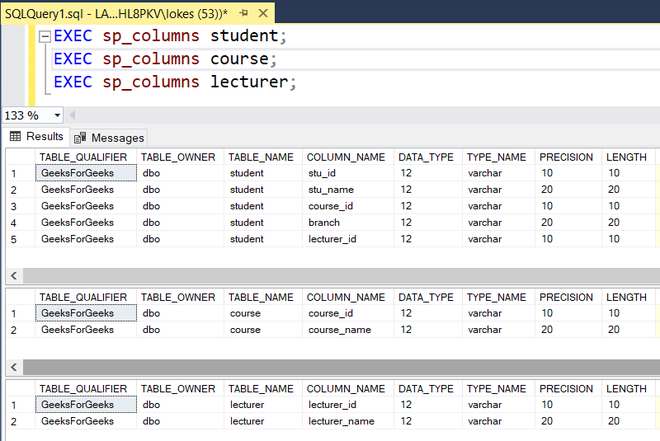### Inserting data into the three tables:

Inserting rows into student table using the following SQL query as follows:

```INSERT INTO student VALUES
('1901401','DEVA','CS1003','C.S', 'P4002'),
('1901402','HARSH','CS1001','C.S', 'P4001'),
('1901403','ABHISHEK','CS1001','C.S', 'P4001'),
('1901404','GARVIT','CS1002','C.S', 'P4003'),
('1901405','SAMPATH','CS1003','C.S', 'P4002'),
('1901406','SATWIK','CS1002','C.S', 'P4003'),
('1901407','GUPTA','CS1001','C.S', 'P4001'),
('1901408','DAS','CS1003','C.S', 'P4002');```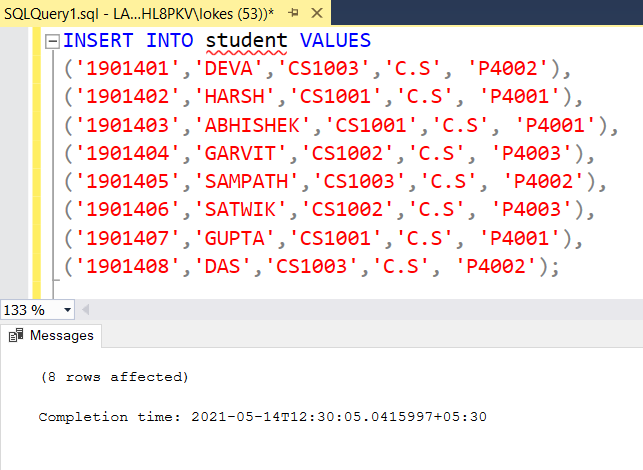Inserting rows into the course table using the following SQL query as follows:

```INSERT INTO course VALUES
('CS1001', 'DBMS'),
('CS1002', 'O.S'),
('CS1003', 'C.N'),
('CS1004', 'M.L'),
('CS1005', 'A.I');```Inserting rows into lecturer table using the following SQL query as follows:

```INSERT INTO lecturer VALUES
('P4001', 'RAMESH'),
('P4002', 'RAVINDER'),
('P4003', 'RAHUL SHARMA'),
('P4005', 'SRINIVASA RAO');```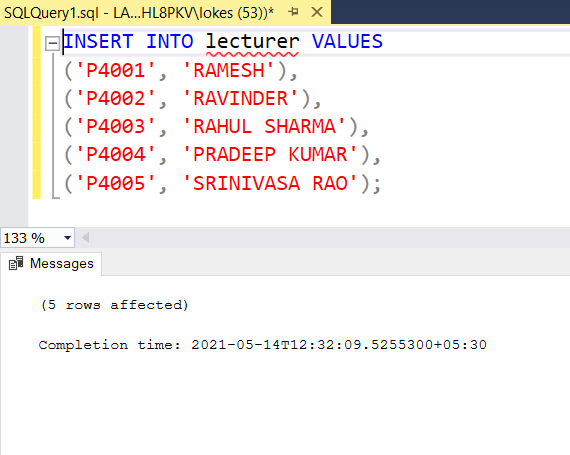### Verifying the inserted data :

Viewing the three tables after inserting rows by using the following SQL query as follows.

```SELECT* FROM student;
SELECT* FROM course;
SELECT* FROM lecturer;```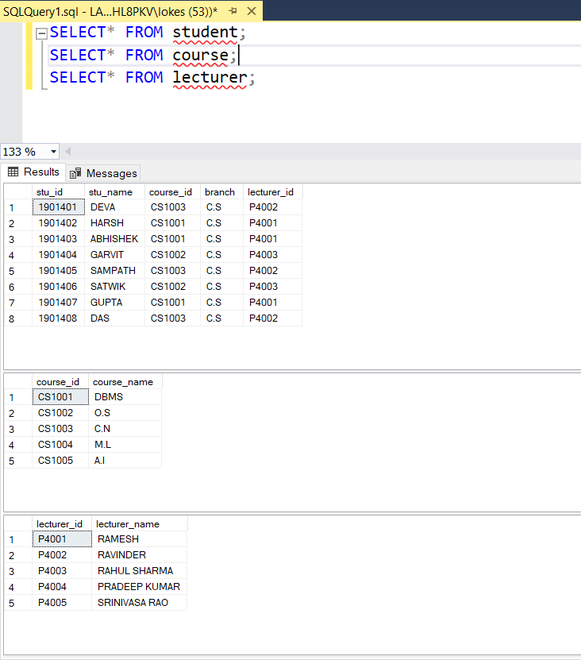### Applying inner joins:

The syntax for multiple joins:

```SELECT column_name1,column_name2,..
FROM table_name1
INNER JOIN
table_name2
ON condition_1
INNER JOIN
table_name3
ON condition_2
INNER JOIN
table_name4
ON condition_3
.
.
.```

Note: While selecting only particular columns use table_name. column_name when there are the same column names in the two tables otherwise you will get an ambiguous error.

### Queries:

Inner Join on two tables student and course:

```SELECT *
FROM student
INNER JOIN
course
ON
student.course_id = course.course_id;```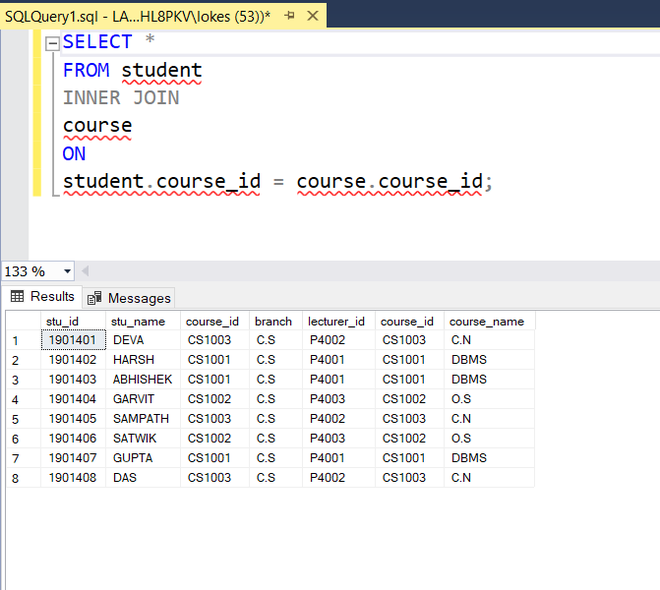All the columns of 2 tables appear that satisfy the equality condition

Inner Join on three tables student, course, and lecturer:

```SELECT *
FROM student
INNER JOIN
course
ON
student.course_id = course.course_id
INNER JOIN
lecturer
ON
student.lecturer_id = lecturer.lecturer_id;```All the columns of 3 tables appear that satisfy the equality condition

Inner join on three tables student, course, lecturer but by selecting particular columns of a particular table.

```SELECT stu_id, stu_name,course.course_id,course.course_name,
lecturer.lecturer_name
FROM student
INNER JOIN
course
ON
student.course_id = course.course_id
INNER JOIN
lecturer
ON
student.lecturer_id = lecturer.lecturer_id;```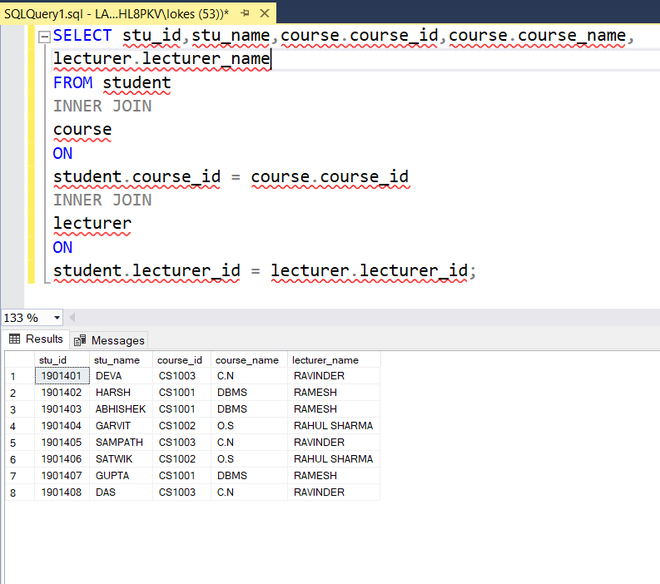My Personal Notes arrow_drop_up